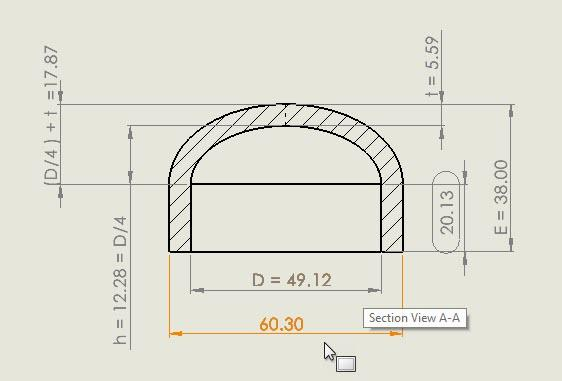# How to determine cylindrical heigth of a ASME B16.9 cap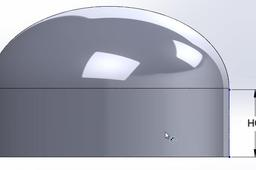How to determine the cylindrical height of an ASME B16.9 cap

1. ### Step 1:

As per ASME B16.9 "The shape of these caps shall be ellipsoidal and shall conform to the shape requirements as given in the ASME Boiler and Pressure Vessel Code."

The table below shows the dimension "E" total height of the caps.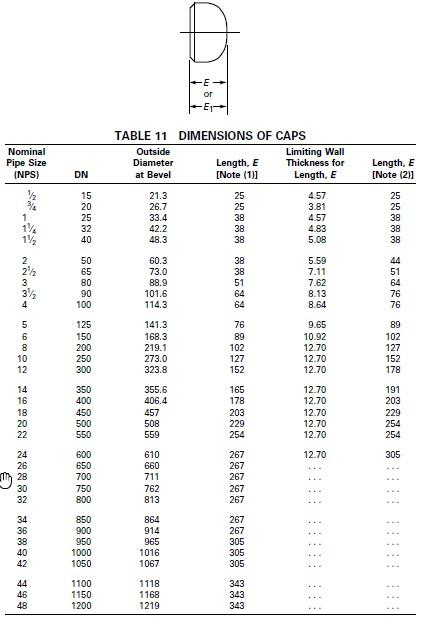2. ### Step 2:

The dimensions can be seen in the image below.

If "t" is the thickness, D = Outer diameter - ( 2 * t)

Then R = D/2.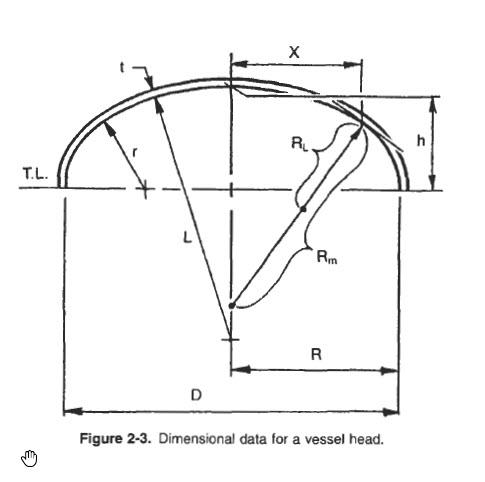3. ### Step 3:

In an ASME ellipsoidal head 2:1, 2:1 is the ratio between

h = R/2 => 2 h = R (2:1)

h = D/4.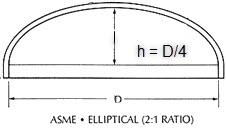4. ### Step 4:

If the total height is E, the cylindrical portion is:

HC = E - ((D/4) + t)

Taken as an example, a cap NPS 2 inch.

External diameter = 60.3 mm ,

Total Heigth = E = 38 mm

D = 60.3 - (2* 5.59 = 49.12 mm

h = D/4 = 12.28 mm

h + t = 17.87

HC = E -(D/4 +t) = 38 -17.87 = 20.13 mm